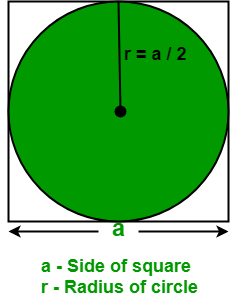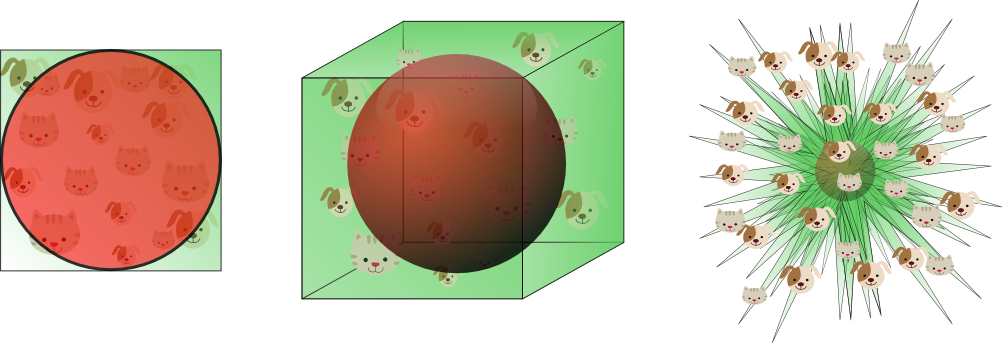# Introduction¶

## 3 Further observations¶

Course: Math 535 - Mathematical Methods in Data Science (MMiDS)
Author: Sebastien Roch, Department of Mathematics, University of Wisconsin-Madison
Updated: Sep 1, 2020

We make a few more observations that will hint at things to come in subsequent topics.

### 3.1 Matrix form of $k$-means clustering¶

The $k$-means clustering objective can be written in matrix form. We will need a notion of matrix norm. A natural way to define a norm for matrices is to notice that an $n \times m$ matrix $A$ can be thought of as an $nm$ vector, with one element for each entry of $A$. Indeed, addition and scalar multiplication work exactly in the same way. Hence, we can define the $2$-norm of a matrix in terms of the sum of its squared entries.

Definition (Frobenius Norm): The Frobenius norm of an $n \times m$ matrix $A \in \mathbb{R}^{n \times m}$ is defined as

$$\|A\|_F = \sqrt{\sum_{i=1}^n \sum_{j=1}^m a_{ij}^2}.$$

$\lhd$

We will encounter other matrix norms later in the course.

As we indicated before, for a collection of $n$ data vectors $\mathbf{x}_1, \ldots, \mathbf{x}_n$ in $\mathbb{R}^d$, it is often convenient to stack them up into a matrix

$$X = \begin{bmatrix} \mathbf{x}_1^T \\ \mathbf{x}_2^T \\ \vdots \\ \mathbf{x}_n^T \\ \end{bmatrix} = \begin{bmatrix} x_{11} & x_{12} & \cdots & x_{1d} \\ x_{21} & x_{22} & \cdots & x_{2d} \\ \vdots & \vdots & \ddots & \vdots \\ x_{n1} & x_{n2} & \cdots & x_{nd} \\ \end{bmatrix}.$$

We can do the same with cluster representatives. Given $\boldsymbol{\mu}_1,\ldots,\boldsymbol{\mu}_k$ also in $\mathbb{R}^d$, we form the matrix

$$U = \begin{bmatrix} \boldsymbol{\mu}_1^T \\ \boldsymbol{\mu}_2^T \\ \vdots \\ \boldsymbol{\mu}_k^T \\ \end{bmatrix} = \begin{bmatrix} \mu_{11} & \mu_{12} & \cdots & \mu_{1d} \\ \mu_{21} & \mu_{22} & \cdots & \mu_{2d} \\ \vdots & \vdots & \ddots & \vdots \\ \mu_{k1} & \mu_{k2} & \cdots & \mu_{kd} \\ \end{bmatrix}.$$

Perhaps less obviously, cluster assignments can also be encoded in matrix form. Recall that, given a partition $C_1,\ldots,C_k$ of $[n]$, we define $c(i) = j$ if $i \in C_j$. For $i=1,\ldots,n$ and $j=1,\ldots,k$, set $z_{ij} = 1$ if $c(i) = j$ and $0$ otherwise, and let $Z$ be the $n \times k$ matrix with entries $z_{ij}$. That is, row $i$ has exactly one entry with value $1$, corresponding to the assigned cluster $c(i)$ of data point $\mathbf{x}_i$, and all other entries $0$.

With this notation, the representative of the cluster assigned to data point $\mathbf{x}_i$ is obtained through the matrix product

$$\boldsymbol{\mu}_{c(i)}^T = \sum_{j = 1}^k z_{ij} \boldsymbol{\mu}_{j}^T = \left(Z U\right)_{i,\cdot}.$$

So

\begin{align*} G(C_1,\ldots,C_k; \boldsymbol{\mu}_1, \ldots, \boldsymbol{\mu}_k) &= \sum_{i=1}^n \|\mathbf{x}_i - \boldsymbol{\mu}_{c(i)}\|^2\\ &= \sum_{i=1}^n \sum_{\ell=1}^d \left(x_{i,\ell} - (Z U)_{i,\ell}\right)^2\\ &= \|X - Z U \|^2_F, \end{align*}

where we used the definition of the Frobenius norm.

In other words, minimizing the $k$-means objective is equivalent to finding a matrix factorization of the form $ZU$ that is a good fit to the data matrix $X$ in Frobenius form. This formulation expresses in a more compact form the idea of representing $X$ as a combination of a small number of representatives. Matrix factorization will come back repeatedly in this course.

NUMERICAL CORNER In Julia, the Frobenius norm of a matrix can be computed using the function norm.

In :
# Julia version: 1.5.1
using Plots, LinearAlgebra, Statistics

In :
A = [1. 0.; 0. 1.; 0. 0.]

Out:
3×2 Array{Float64,2}:
1.0  0.0
0.0  1.0
0.0  0.0
In :
norm(A)

Out:
1.4142135623730951

### 3.2 High-dimensional space¶

Applying Chebyshev's inequality to sums of independent random variables has useful statistical implications: it shows that, with a large enough number of samples $n$, the sample mean is close to the population mean. Hence it allows us to infer properties of a population from samples. Interestingly, one can apply a similar argument to a different asymptotic regime: the limit of large dimension $d$. But as we will see in this section, the statistical implications are quite different.

#### 3.2.1 High-dimensional cube¶

To start explaining the quote above, we consider a simple experiment. Let $\mathcal{C} = [-1/2,1/2]^d$ be the $d$-cube with side lengths $1$ centered at the origin and let $\mathcal{B} = \{\mathbf{x} \in \mathbb{R}^d : \|x\|\leq 1/2\}$ be the inscribed $d$-ball. In $d=2$ dimensions:(Source)

Now pick a point $\mathbf{X}$ uniformly at random in $\mathcal{C}$. What is the probability that it falls in $\mathcal{B}$?

To generate $\mathbf{X}$, we pick $d$ independent random variables $X_1, \ldots, X_d \sim \mathrm{U}[-1/2, 1/2]$, and form the vector $\mathbf{X} = (X_1, \ldots, X_d)$. Indeed, the PDF of $\mathbf{X}$ is then $f_{\mathbf{X}}(\mathbf{x})= 1^d = 1$ if $\mathbf{x} \in \mathcal{C}$ and $0$ otherwise.

The event we are interested in is $A = \left\{\|\mathbf{X}\| \leq 1/2\right\}$. The uniform distribution over the set $\mathcal{C}$ has the property that $\mathbb{P}[A]$ is the volume of $A$ divided by the volume of $\mathcal{C}$. In this case, the volume of $\mathcal{C}$ is $1^d = 1$ and the volume of $A$ has an explicit formula.

This leads to the following surprising fact:

Theorem (High-dimensional Cube) Let $\mathcal{B} = \{\mathbf{x} \in \mathbb{R}^d \,:\, \|\mathbf{x}\|\leq 1/2\}$ and $\mathcal{C} = [-1/2,1/2]^d$. Pick $\mathbf{X} \sim \mathrm{U}[\mathcal{C}]$. Then, as $d \to +\infty$,

$$\mathbb{P}[\mathbf{X} \in \mathcal{B}] \to 0.$$

In words, in high dimension if one picks a point at random from the cube, it is unlikely to be close to the origin. Instead it is likely to be in the corners. A geometric interpretation is that a high-dimensional cube is a bit like a spiky ball. A visualization of this theorem:(Source)

We give a proof based on Chebyshev's inequality. It has the advantage of providing some insight into this counter-intuitive phenomenon by linking it to the concentration of sums of independent random variables, in this case the squared norm of $\mathbf{X}$.

Proof idea: We think of $\|\mathbf{X}\|^2$ as a sum of independent random variables and apply Chebyshev's inequality. It implies that the norm of $\mathbf{X}$ is concentrated around its mean, which grows like $\sqrt{d}$. The latter is larger than $1/2$ for $d$ large.

Proof: Write out $\|\mathbf{X}\|^2 = \sum_{i=1}^d X_i^2$. Using linearity of expectation and the fact that the $X_i$'s are independent, we get

$$\mathbb{E}\left[ \|\mathbf{X}\|^2 \right] = \sum_{i=1}^d \mathbb{E}[X_i^2] = d \,\mathbb{E}[X_1^2]$$

and

$$\mathrm{Var}\left[ \|\mathbf{X}\|^2 \right] = \sum_{i=1}^d \mathrm{Var}[X_i^2] = d \,\mathrm{Var}[X_1^2].$$

We bound the probability of interest as follows. We first square the norm and center around the mean:

\begin{align} \mathbb{P} \left[ \|\mathbf{X}\| \leq 1/2 \right] &= \mathbb{P} \left[ \|\mathbf{X}\|^2 \leq 1/4 \right]\\ &= \mathbb{P} \left[ \|\mathbf{X}\|^2 - \mathbb{E}[\|\mathbf{X}\|^2]\leq 1/4 - d \,\mathbb{E}[X_1^2] \right]. \end{align}

Now notice that $\mathbb{E}[X_1^2] > 0$ does not depend on $d$. Take $d$ large enough that $d \,\mathbb{E}[X_1^2] > 1/4$. We then use the following fact: if $\alpha = d \mathbb{E}[X_1^2] - 1/4 > 0$ and $Z = \|X\|^2 - \mathbb{E}\|X\|^2$, we can write by monotonicity and the definition of the absolute value

$$\mathbb{P}[Z \leq -\alpha] \leq \mathbb{P}[Z \leq -\alpha\text{ or }Z \geq \alpha] = \mathbb{P}[|Z| \geq \alpha].$$

We arrive at

$$\mathbb{P} \left[ \|\mathbf{X}\| \leq 1/2 \right] \leq \mathbb{P} \left[ \left| \|\mathbf{X}\|^2 - \mathbb{E}[\|\mathbf{X}\|^2] \right| \geq d \,\mathbb{E}[X_1^2] - 1/4 \right].$$

We can now apply Chebyshev's inequality to the right-hand side, which gives

\begin{align} \mathbb{P} \left[ \|\mathbf{X}\| \leq 1/2 \right] &\leq \frac{\mathrm{Var}\left[ \|\mathbf{X}\|^2 \right]} {(d \,\mathbb{E}[X_1^2] - 1/4)^2}\\ &= \frac{d \,\mathrm{Var}[X_1^2]} {(d \,\mathbb{E}[X_1^2] - 1/4)^2}\\ &= \frac{1}{d} \cdot \frac{\mathrm{Var}[X_1^2]} {(\mathbb{E}[X_1^2] - 1/(4d))^2} . \end{align}

Again, $\mathrm{Var}[X_1^2]$ does not depend on $d$. So the right-hand side goes to $0$ as $d \to +\infty$, as claimed.$\square$

We will see later in the course that this high-dimensional phenomenon has implications for data science problems. It is behind what is referred to as the Curse of Dimensionality.

NUMERICAL CORNER We can check the theorem in a simulation. Here we pick $n$ points uniformly at random in the $d$-cube $\mathcal{C}$, for a range of dimensions $[d_\min, d_\max]$. We then plot the frequency of landing in the inscribed $d$-ball $\mathcal{B}$ and see that it rapidly converges to $0$. Alternatively, we could just plot the formula for the volume of $\mathcal{B}$. But knowing how to do simulations is useful in situations where explicit formulas are unavailable or intractable.

In :
function highdim_cube(dmax, n)
in_ball = zeros(Float64, dmax) # in-ball freq
for d=1:dmax # for each dimension
in_ball[d] = mean([(norm(rand(d) .- 1/2) < 1/2) for i=1:n])
end
plot(1:dmax, in_ball,
legend=false, markershape=:diamond, xlabel="dim", ylabel="in-ball freq")
end

Out:
highdim_cube (generic function with 1 method)
In :
highdim_cube(10, 1000)

Out:

#### 3.2.2 Gaussians in high dimension [optional]¶

In this optional section, we turn our attention to the Gaussian (or Normal) distribution and its behavior in high dimension. Using Chebyshev's inequality, we show that a standard Normal vector has the following counter-intuititve property in high dimension: a typical draw has $2$-norm that is highly likely to be around $\sqrt{d}$. Visually, when $d$ is large, the joint PDF looks something like this:(Source)

This is unexpected because the joint PDF is maximized at $\mathbf{x} = \mathbf{0}$ for all $d$ (including $d=1$ as can be seen in the figure above). But the rough intuition is the following: (1) there is only "one way" to obtain $\|\mathbf{X}\|^2 = 0$ -- every coordinate must be $0$ by the point-separating property of the $2$-norm; (2) on the other hand, there are "many ways" to obtain $\|\mathbf{X}\|^2 = \sqrt{d}$ -- and that compensates for the lower density.

Theorem (High-dimensional Gaussians) Let $\mathbf{X}$ be a standard Normal $d$-vector. Then, for any $\varepsilon > 0$,

$$\mathbb{P} \left[\, \|\mathbf{X}\| \notin (\sqrt{d(1-\varepsilon)}, \sqrt{d(1+\varepsilon)}) \,\right] \to 0$$

as $d \to +\infty$.

Proof idea: We apply Chebyshev's inequality to the squared norm, which is a sum of independent random variables.

Proof: Let $Z = \|\mathbf{X}\|^2 = \sum_{i=1}^d X_i^2$ and notice that, by definition, it is a sum of independent random variables. Appealing to the expectation and variance formulas from the previous sections:

$$\mathbb{E}[\|\mathbf{X}\|^2] = d \,\mathbb{E}[X_1^2] = d \,\mathrm{Var}[X_1] = d$$

and

$$\mathrm{Var}[\|\mathbf{X}\|^2] = d \,\mathrm{Var}[X_1^2]$$

where $\mathrm{Var}[X_1^2]$ does not depend on $d$. By Chebyshev's inequality

$$\mathbb{P}\left[ \|\mathbf{X}\|^2 \notin \left( d(1-\varepsilon),d(1+\varepsilon) \right) \right] = \mathbb{P}[|\|\mathbf{X}\|^2 - d| \geq \varepsilon d] \leq \frac{d \,\mathrm{Var}[X_1^2]}{\varepsilon^2 d^2} = \frac{\mathrm{Var}[X_1^2]}{d \varepsilon^2}.$$

Taking a square root inside the probability on the leftmost side and taking a limit as $d \to +\infty$ on the rightmost side gives the claim.$\square$

NUMERICAL CORNER We check our claim in a simulation. We generate standard Normal $d$-vectors using the randn function and plot the histogram of their $2$-norm.

In :
function normal_shell(d, n)
one_sample_norm = [norm(randn(d)) for i=1:n]
histogram(one_sample_norm,
legend=false, xlims=(0,maximum(one_sample_norm)), nbin=20)
end

Out:
normal_shell (generic function with 1 method)
In :
normal_shell(1, 10000)

Out:

In higher dimension:

In :
normal_shell(100, 10000)

Out: